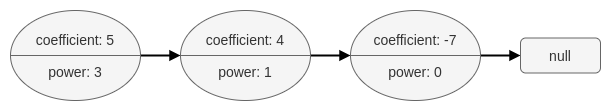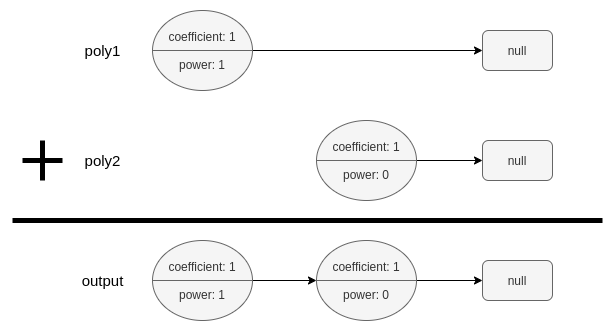Formatted question description: https://leetcode.ca/all/1634.html

# 1634. Add Two Polynomials Represented as Linked Lists

Medium

## Description

A polynomial linked list is a special type of linked list where every node represents a term in a polynomial expression.

Each node has three attributes:

• coefficient: an integer representing the number multiplier of the term. The coefficient of the term 9x^4 is 9.
• power: an integer representing the exponent. The power of the term 9x^4 is 4.
• next: a pointer to the next node in the list, or null if it is the last node of the list.

For example, the polynomial 5x^3 + 4x - 7 is represented by the polynomial linked list illustrated below:The polynomial linked list must be in its standard form: the polynomial must be in strictly descending order by its power value. Also, terms with a coefficient of 0 are omitted.

Given two polynomial linked list heads, poly1 and poly2, add the polynomials together and return the head of the sum of the polynomials.

PolyNode format:

The input/output format is as a list of n nodes, where each node is represented as its [coefficient, power]. For example, the polynomial 5x^3 + 4x - 7 would be represented as: [[5,3],[4,1],[-7,0]].

Example 1:Input: poly1 = [[1,1]], poly2 = [[1,0]]

Output: [[1,1],[1,0]]

Explanation: poly1 = x. poly2 = 1. The sum is x + 1.

Example 2:

Input: poly1 = [[2,2],[4,1],[3,0]], poly2 = [[3,2],[-4,1],[-1,0]]

Output: [[5,2],[2,0]]

Explanation: poly1 = 2x2 + 4x + 3. poly2 = 3x2 - 4x - 1. The sum is 5x2 + 2. Notice that we omit the “0x” term.

Example 3:

Input: poly1 = [[1,2]], poly2 = [[-1,2]]

Output: []

Explanation: The sum is 0. We return an empty list.

Constraints:

• 0 <= n <= 10^4
• -10^9 <= PolyNode.coefficient <= 10^9
• PolyNode.coefficient != 0
• 0 <= PolyNode.power <= 10^9
• PolyNode.power > PolyNode.next.power

## Solution

Create a dummyHead for the result polynomial. Each time compare the current nodes of poly1 and poly2, which are node1 and node2.

If they have the same power, calculate the sum of the coefficients of node1 and node2. If the sum is not 0, append it to the result polynomial, move the node of the result polynomial one step ahead. Otherwise, do not append it to the result polynomial. Move node1 and node2 one step ahead.

If they have different powers, append the node with the greater power to the result polynomial, move the node of the result polynomial one step ahead, and move the corresponding node (with the greater power) one step ahead.

When one of the nodes in node1 and node2 becomes empty, append the remaining nodes of the other polynomial to the result node.

Finally, return dummyHead.next.

/**
* Definition for polynomial singly-linked list.
* class PolyNode {
*     int coefficient, power;
*     PolyNode next = null;

*     PolyNode() {}
*     PolyNode(int x, int y) { this.coefficient = x; this.power = y; }
*     PolyNode(int x, int y, PolyNode next) { this.coefficient = x; this.power = y; this.next = next; }
* }
*/

class Solution {
public PolyNode addPoly(PolyNode poly1, PolyNode poly2) {
PolyNode dummyHead = new PolyNode();
PolyNode node = dummyHead;
PolyNode node1 = poly1, node2 = poly2;
while (node1 != null && node2 != null) {
if (node1.power == node2.power) {
int nextCoefficient = node1.coefficient + node2.coefficient;
int nextPower = node1.power;
if (nextCoefficient != 0) {
PolyNode nextNode = new PolyNode(nextCoefficient, nextPower);
node.next = nextNode;
node = node.next;
}
node1 = node1.next;
node2 = node2.next;
} else if (node1.power > node2.power) {
PolyNode nextNode = new PolyNode(node1.coefficient, node1.power);
node.next = nextNode;
node = node.next;
node1 = node1.next;
} else {
PolyNode nextNode = new PolyNode(node2.coefficient, node2.power);
node.next = nextNode;
node = node.next;
node2 = node2.next;
}
}
if (node1 != null)
node.next = node1;
else if (node2 != null)
node.next = node2;